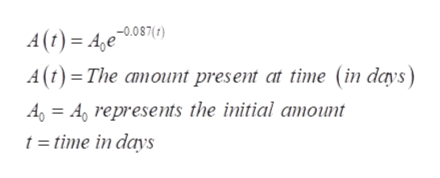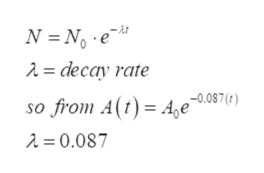# Iodine 131 is a radioactive material that decays according to the function A(t)=A0e-0.087(t) where A0 is the initial amount present, and A is the amount present at time (in days) Assume that a scientist has a sample of 200 grams of iodine 131.What is the decay rate of iodine 131?How much iodine 132 is left after 8 days?When will 70 grams of iodine 131 be left?What is the half-life of iodine 131?

Question
53 views

Iodine 131 is a radioactive material that decays according to the function A(t)=A0e-0.087(t) where A0 is the initial amount present, and A is the amount present at time (in days) Assume that a scientist has a sample of 200 grams of iodine 131.

1. What is the decay rate of iodine 131?
2. How much iodine 132 is left after 8 days?
3. When will 70 grams of iodine 131 be left?
4. What is the half-life of iodine 131?
check_circle

Step 1

Refer to the question . we have been given the iodine 131 which is a radioactive material that decays according to the function A(t)=A0e-0.087(t) . Here A0 represents the initial amount present and A is the amount present at time (in days). Also the sample is provided with 200 grams of iodine 131help_outlineImage TranscriptioncloseA(t) 4e0870) A (t) The amount present at time (in days) A= Arepresents the initial amount t=time in days fullscreen
Step 2

(1)…To find the decay rate of iodine 131 compare the provided equation with the radioactive decay equation and from there we can find the decay rate as 0.087help_outlineImage TranscriptioncloseN No6 = decay rate so from A(t) = A e-0.07() A = 0.087 fullscreen
Step 3

(2)…

As we can find the amount of iodine 131 and not 132 because we have not given the information about 132 ...

### Want to see the full answer?

See Solution

#### Want to see this answer and more?

Solutions are written by subject experts who are available 24/7. Questions are typically answered within 1 hour.*

See Solution
*Response times may vary by subject and question.
Tagged in

### Other